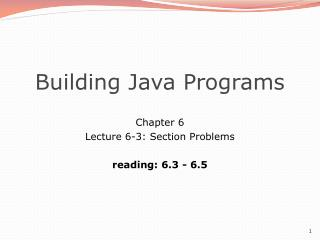Download PresentationBuilding Java Programs

# Building Java Programs - PowerPoint PPT PresentationDownload Presentation## Building Java Programs

- - - - - - - - - - - - - - - - - - - - - - - - - - - E N D - - - - - - - - - - - - - - - - - - - - - - - - - - -
##### Presentation Transcript

1. Building Java Programs Chapter 6 Lecture 6-3: Section Problems reading: 6.3 - 6.5

2. Recall: Line-based methods nextLine consumes from the input cursor to the next \n . Scanner input = new Scanner(new File("<filename>")); while (input.hasNextLine()) { String line = input.nextLine(); <process this line>; }

3. What's wrong with this? public class Hours { public static void main(String[] args) throws FileNotFoundException { Scanner input = new Scanner(new File("hours.txt")); while (input.hasNextLine()) { String line = input.nextLine(); int id = line.nextInt(); // e.g. 456 String name = line.nextLine(); // e.g. "Greg" double sum = 0.0; int count = 0; while (line.hasNextDouble()) { sum = sum + line.nextDouble(); count++; } double average = sum / count; System.out.println(name + " (ID#" + id + ") worked " + sum + " hours (" + average + " hours/day)"); } } }

4. Corrected code public class Hours { public static void main(String[] args) throws FileNotFoundException { Scanner input = new Scanner(new File("hours.txt")); while (input.hasNextLine()) { String line = input.nextLine(); Scanner tokens = new Scanner(line); int id = tokens.nextInt(); // e.g. 456 String name = tokens.next(); // e.g. "Greg" double sum = 0.0; int count = 0; while (tokens.hasNextDouble()) { sum = sum + tokens.nextDouble(); count++; } double average = sum / count; System.out.println(name + " (ID#" + id + ") worked " + sum + " hours (" + average + " hours/day)"); } } }

5. Recall: Tokenizing lines A String Scanner can tokenize each line of a file. Scanner input = new Scanner(new File("filename")); while (input.hasNextLine()) { String line = input.nextLine(); Scanner tokens = new Scanner(line); <process the tokens on this line>; }

6. collapseSpaces exercise • Write a method collapeSpaces that accepts a file Scanner and writes that file's text to the console, with multiple spaces/tabs between words reduced to a single space.

7. Exercise solution public static void collapseSpaces(Scanner input) { while (input.hasNextLine()) { String line = input.nextLine(); Scanner words = new Scanner(line); if (words.hasNext()) { System.out.print(words.next()); while (words.hasNext()) { System.out.print(" " + words.next()); } } System.out.println(); } }

8. leetSpeak exercise • Write a method leetSpeak that accepts two parameters: • a Scanner representing an input file, and • a PrintStream representing an output file. • Convert the input file's text to "leet speak", where various letters are replaced by other letters/numbers. Output the leet version of the text to the given output file. • Preserve the original line breaks from the input. • Wrap each word of input in parentheses. // example call Scanner input = new Scanner(new File("lincoln.txt")); PrintStream output = new PrintStream(new File("leet.txt")); leetSpeak(input, output);

9. 1337-speak replacements

10. Exercise solution public static void leetSpeak(Scanner input, PrintStream output) { while (input.hasNextLine()) { String line = input.nextLine(); Scanner lineScanner = new Scanner(line); while (lineScanner.hasNext()) { String word = lineScanner.next(); word = word.replace("o", "0"); word = word.replace("l", "1"); word = word.replace("e", "3"); word = word.replace("a", "4"); word = word.replace("t", "7"); if (word.endsWith("s")) { word = word.substring(0, word.length() - 1) + "Z"; } output.print("(" + word + ") "); } output.println(); // preserve line breaks } }

11. Mixing tokens and lines Using nextLine in conjunction with the token-based methods on the same Scanner can cause bad results. 23 3.14Joe "Hello world" 45.2 19 You'd think you could read 23 and 3.14 with nextInt and nextDouble, then read Joe "Hello world" with nextLine . System.out.println(input.nextInt()); // 23 System.out.println(input.nextDouble()); // 3.14 System.out.println(input.nextLine()); // But the nextLine call produces no output! Why?

12. Mixing lines and tokens Don't read both tokens and lines from the same Scanner: 23 3.14Joe "Hello world" 45.2 19 input.nextInt() // 2323\t3.14\nJoe\t"Hello world"\n\t\t45.2 19\n ^ input.nextDouble() // 3.1423\t3.14\nJoe\t"Hello world"\n\t\t45.2 19\n ^ input.nextLine() // "" (empty!)23\t3.14\nJoe\t"Hello world"\n\t\t45.2 19\n ^ input.nextLine() // "Joe\t\"Hello world\""23\t3.14\nJoe\t"Hello world"\n\t\t45.2 19\n ^

13. Line-and-token example Scanner console = new Scanner(System.in); System.out.print("Enter your age: "); int age = console.nextInt(); System.out.print("Now enter your name: "); String name = console.nextLine(); System.out.println(name + " is " + age + " years old."); Log of execution (user input underlined): Enter your age: 12 Now enter your name: Sideshow Bob is 12 years old. Why? Overall input: 12\nSideshow Bob After nextInt(): 12\nSideshow Bob ^ After nextLine(): 12\nSideshow Bob ^